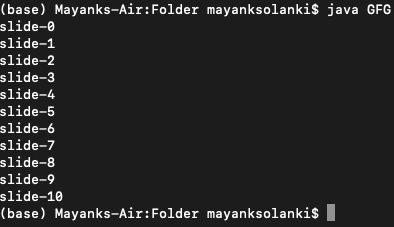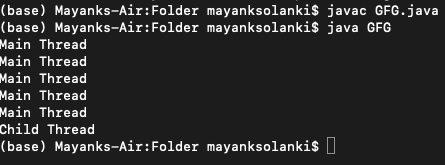# Difference Between sleep and yield Method in Java

• Last Updated : 09 Jun, 2021

In java if a thread doesn’t want to perform any operation for a particular amount of time then we should go for the sleep() method, which causes the currently executing thread to stop for the specified number of milliseconds.

Syntax :

```public static native void sleep( long ms) throws InterruptedException ;
// The above method put the thread in sleep for a specified number of millisecond```
```public static void sleep(long ms , int ns) throws InterruptedException
// The above method also put the thread in sleep for a specified number of milliseconds
// plus specified number of nanoseconds```

Method 1: sleep() method

Every sleep() method in java throws an InterruptedException which is a checked exception hence whenever we are using the sleep method compulsory we should handle it either by try-catch or by throws keyword otherwise we will get compile-time error.

Implementation: Here, we put the main thread in sleep for 5second after the “println” statement. So every slide takes 5second to print.

Example

## Java

 `// Java Program to illustrate sleep method`   `// Importing all input output classes` `import` `java.io.*;` `// Importing all utility classes from` `// java.util package` `import` `java.util.*;`   `// Main class` `class` `GFG {`   `    ``// Main driver method` `    ``public` `static` `void` `main(String[] args)` `        ``throws` `InterruptedException` `    ``{`   `        ``// Iterating using simple for loops` `        ``for` `(``int` `i = ``0``; i <= ``10``; i++) {`   `            ``// Printing the current thread slide` `            ``System.out.println(``"slide-"` `+ i);`   `            ``// Putting the main thread to sleep for 5 second` `            ``Thread.sleep(``5000``);` `        ``}`   `        ``// Here after every  5 seconds a slide will be` `        ``// printed` `    ``}` `}`

Output:Note: Here slide-1 will be printed after slide-0 after 5000 nanoseconds so do apply for the rest of all the slides. Hence this output will be displayed taking a certain time in execution in the runtime state.

Method 2: yield() method

It causes to pause the currently executing thread to give the chance for waiting thread of same priority. If there are no waiting threads or all waiting threads have low priority then the same thread can continue its execution. If multiples thread is waiting with same priority then which waiting thread will get the chance we can’t say it depends on the thread scheduler. The thread which is yield when it will get the chance once again also depends on the thread scheduler.

Syntax:

`public static native void yield( );`

Implementation:

Example

## Java

 `// Java Program to illustrate yield() method`   `// Importing input output classes` `import` `java.io.*;` `// Importing all utility classes` `import` `java.util.*;`   `// Class 1` `// Helper class extending Thread class`   `// Creating a thread in our myThread class` `// by extending the Thread class`   `class` `myThread ``extends` `Thread {`   `    ``// Method in helper class` `    ``// Declaring run method` `    ``public` `void` `run()` `    ``{`   `        ``// Displaying the message` `        ``System.out.println(``"Child Thread"``);`   `        ``// Calling yield() method` `        ``Thread.yield();` `    ``}` `}`   `// Class 2` `// Main class` `class` `GFG {`   `    ``// Main driver method` `    ``public` `static` `void` `main(String[] args)` `        ``throws` `InterruptedException` `    ``{`   `        ``// Creating a thread object in the main() method` `        ``// of our helper class above` `        ``myThread t = ``new` `myThread();`   `        ``// Starting the above thread created` `        ``// using the start() method` `        ``t.start();`   `        ``// Iterating using for loop` `        ``// over custom taken size equals 5` `        ``for` `(``int` `i = ``0``; i < ``5``; i++) {` `            ``// Display message` `            ``System.out.println(``"Main Thread"``);` `        ``}` `    ``}` `}`Output explanation:

In the above program if we are commenting the line Thread.yield() both threads will be executed simultaneously, and we can’t expect which thread will get completed first. If we are not commenting Thread.yield() method then because of that main thread will get a chance more times and the chance of completing the main thread first is high.

So finally we are done with both the methods, let s finally conclude the differences between them.

My Personal Notes arrow_drop_up
Recommended Articles
Page :